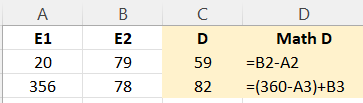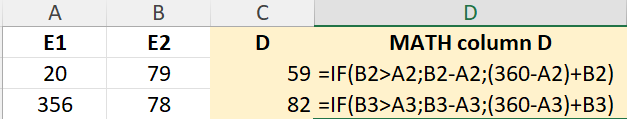# mathematical calculations with if loop

good evening
I would need to know the node that allows me to perform the following calculation in column D, see excel table.
In excel i would use the function if, but i would like to implement a work already started in knimeI would also need to insert a new column with a numeric value

Best regards

Luigi

Hello @lriva ,

for the formulas in column D you can use a Rule Engine node. Sintax is simple:
if this condition is true => then apply this formula
else if this condition is true => then apply this other formula

For the new column, if the value is the same for all rows, use a constant value column. If it’s based on a condition, use a rule engine once again. Otherwise, insert it in the Excel and import it in KNIME.

Have a nice evening,
Raffaello

Hi @lriva
I don’t fully understand about the logic IF in the picture.
But I would probably use the ‘Math Formula’ node. The syntax is very similar to Excel; If-Condition:

`if (cond, trueval, falseval)`

You can concatenate conditions in the same way that you do in Excel.

BR

3 Likes

Hi @lelloba

Rule Engine does not allow “apply this formula”. You can only either assign a static value, or the value of a column/variable. You can’t do operations (or String manipulations) in Rule Engine.

For Math operations, @gonhaddock is correct, there is the `if (cond, trueval, falseval)`, although, like @gonhaddock , I cannot see where the IF is needed based on the screenshot from Excel.

It looks again like another case where the requester gave the minimum information. Please “help us help you. The more details you give, the more precise the solution will be”. I’m quoting this because it’s something I keep repeating.

4 Likes

You are right, @bruno29a, Sorry for the mistakeRaffaello

1 Like

thanks for the answer now I will try.
I thought with the excel table screen it was clear my “problem-support request”.
I had entered in columns A and B the input data and in column C the result I should get and in column D the mathematical formula. I thought it was clear but maybe this new diagram is better.In any case thanks for the information so far, I will try now

you are great for the support you give

Luigi

3 Likes

I succeeded!
thank you

1 Like

This topic was automatically closed 90 days after the last reply. New replies are no longer allowed.